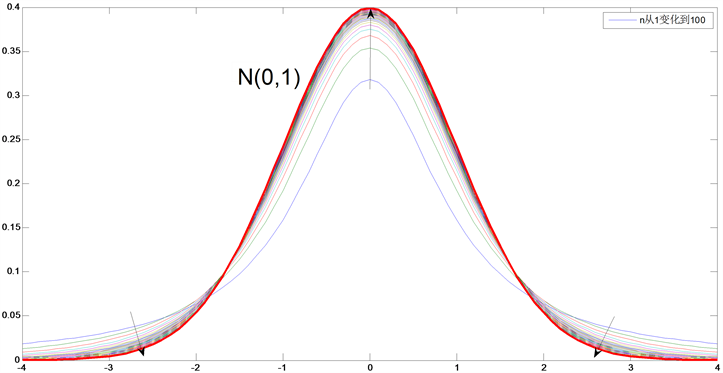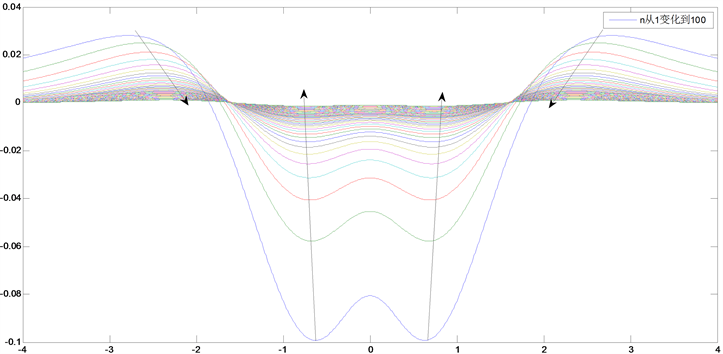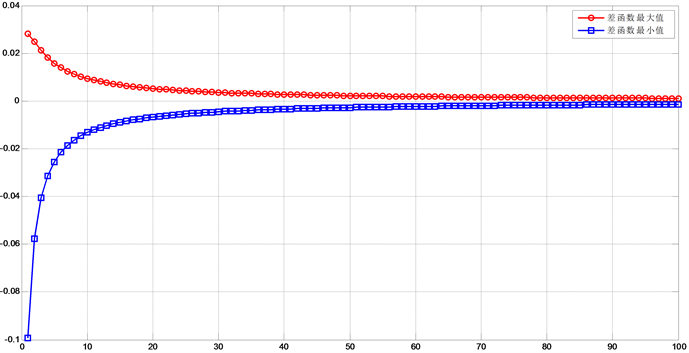﻿ t分布的渐进正态性证明及其特点分析

# t分布的渐进正态性证明及其特点分析Proof of Asymptotic Normality of t Distribution and Analysis of Its Characteristics

Abstract: Previous studies have proved that the t distribution function has the characteristics of asymptotic normality. This paper applies the characteristics of the difference function to prove the asymptotic normality of the t distribution again, improving the previous research. And with the use of MATLAB software, the asymptotic normality is verified intuitively. After that, we further analyze the char-acteristics of the t function with the help of intuitive graphics.

1. 引言

2. t分布及其性质

2.1. t分布的定义

${f}_{t}\left(x,n\right)=\frac{\Gamma \left(\frac{n+1}{2}\right)}{\sqrt{n\pi }\Gamma \left(\frac{n}{2}\right)}{\left(1+\frac{{x}^{2}}{n}\right)}^{-\frac{n+1}{2}}$, $\left(-\infty (1)

2.2. t分布的性质

${\zeta }_{1}~N\left(0,1\right),{\zeta }_{2}~N\left(0,1\right),\cdots ,{\zeta }_{n}~N\left(0,1\right)$，那么随机变量 $X=\frac{\xi }{\sqrt{\underset{i=1}{\overset{n}{\sum }}{\zeta }_{i}/n}}$，服从自由度为n的t分布；

3. 利用差函数分析证明渐进正态性

t分布函数与标准正态分布的差函数的意义为两者在y轴方向的距离，若当n趋于无穷时，差函数在其各极值点的取值均趋于0，则可间接证明当n趋于无穷时，t分布趋于标准正态分布，即

$\underset{n\to \infty }{\mathrm{lim}}\mathrm{sup}\phi \left(x,n\right)=0$，则 $t\left(n\right)$ 趋近标准正态分布 $N\left(0,1\right)$

3.1. 差函数的定义

${f}_{t}\left(x,n\right)=\frac{\Gamma \left(\frac{n+1}{2}\right)}{\sqrt{n\pi }\Gamma \left(\frac{n}{2}\right)}{\left(1+\frac{{x}^{2}}{n}\right)}^{-\frac{n+1}{2}}$ (2)

${f}_{t}\left(x,n\right)=\frac{\Gamma \left(\frac{n+1}{2}\right)}{\sqrt{n\pi }\Gamma \left(\frac{n}{2}\right)}{\left(1+\frac{{x}^{2}}{n}\right)}^{-\frac{n+1}{2}}$ (3)

3.2. 差函数的基本性质

$\frac{\text{d}\phi \left(x,n\right)}{\text{d}x}=x\left[\frac{n+1}{n}\frac{\Gamma \left(\frac{n+1}{2}\right)}{\sqrt{n\pi }\Gamma \left(\frac{n}{2}\right)}{\left(1+\frac{{x}^{2}}{n}\right)}^{-\frac{n+3}{2}}-\frac{1}{\sqrt{2\pi }}{\text{e}}^{-\frac{{x}^{2}}{2}}\right]$ (4)

$\frac{\text{d}\phi \left(x,n\right)}{\text{d}x}=0$ 得到极值点 ${x}_{0}=0$，另有极值点 ${x}_{k}$ 满足方程

$\frac{n+1}{n}\frac{\Gamma \left(\frac{n+1}{2}\right)}{\sqrt{n\pi }\Gamma \left(\frac{n}{2}\right)}{\left(1+\frac{{x}^{2}}{n}\right)}^{-\frac{n+3}{2}}=\frac{1}{\sqrt{2\pi }}{\text{e}}^{-\frac{{x}^{2}}{2}}$ (5)

$\phi \left({x}_{k},n\right)=\frac{\Gamma \left(\frac{n+1}{n}\right)}{\sqrt{n\pi }\Gamma \left(\frac{n}{2}\right)}{\left(1+\frac{{x}_{k}}{n}\right)}^{-\frac{n+1}{2}}\left[1-\frac{n+1}{n}{\left(1+\frac{{x}_{k}^{2}}{n}\right)}^{-1}\right]$ (6)

3.3. t分布的正态渐进性证明

$\underset{n\to \infty }{\mathrm{lim}}\frac{\left[\frac{\left(2n-1\right)!!}{\left(2n\right)!!}\right]}{\sqrt{n\pi }}=1\text{\hspace{0.17em}}\text{\hspace{0.17em}}\text{\hspace{0.17em}}\left(n\in N\right)$ (7)

$\underset{n=1}{\overset{\infty }{\prod }}\frac{2n}{2n-1}×\frac{2n}{2n+1}=\frac{\pi }{2}\text{\hspace{0.17em}}\text{\hspace{0.17em}}\text{\hspace{0.17em}}\left(n\in N\right)$ (8)

$\underset{n\to \infty }{\mathrm{lim}}{\left[\frac{\left(2n\right)!!}{\left(2n-1\right)!!}\right]}^{2}\frac{1}{2n-1}=\frac{\pi }{2}$ (9)

$\begin{array}{l}\underset{n\to \infty }{\mathrm{lim}}\left[\frac{\left(2n\right)!!}{\left(2n-1\right)!!}\right]\sqrt{\frac{2}{\left(2n-1\right)\pi }}=1\\ \therefore \underset{n\to \infty }{\mathrm{lim}}\left[\frac{\left(2n\right)!!}{\left(2n-1\right)!!}\right]\sqrt{\frac{1}{n\pi }}=1\end{array}$ (10)

$\underset{n\to \infty }{\mathrm{lim}}\phi \left({x}_{0},n\right)=\frac{\Gamma \left(\frac{n+1}{n}\right)}{\sqrt{n\pi }\Gamma \left(\frac{n}{2}\right)}-\frac{1}{\sqrt{2\pi }}$ (11)(12)(13)

$\underset{n\to \infty }{\mathrm{lim}}{f}_{t}\left({x}_{k},n\right)=p$, (p为一有限数) (14)

$\underset{n\to \infty }{\mathrm{lim}}\left[1-\frac{n+1}{n}{\left(1+\frac{{x}_{k}^{2}}{n}\right)}^{-1}\right]=0$ (15)

$\underset{n\to \infty }{\mathrm{lim}}\phi \left({x}_{k},n\right)=0$ (16)

4. t分布函数的渐进正态性直观验证

clear all;clc;

x=-4:0.1:4;

n=linspace(1,100,100);

axis([-4 4 0 0.41]);

ylabel('$t(n)$','interpreter','latex', 'FontSize', 18);

xlabel('x')

for i=1:100

A(i,:)=tpdf(x,n(i));

end

plot(x,A);

hold on;

z=normpdf(x,0,1)

plot(x,z,'color','r','linewidth',2.3);

title('自由度从1到100的t分布密度函数和标准正态分布');

legend('n从1变化到100');Figure 1. Asymptotic normality of t distribution

5. 利用差函数分析其具体特点

5.1. 差函数的变化图像

clc;clear all;

x=-4:0.01:4;

n=linspace(1,100);

axis([-4 4 -0.2 0.41]);

ylabel('$t(n)-N(0,1)$','interpreter','latex', 'FontSize', 18);

xlabel('x')

z=normpdf(x,0,1)

for i=1:100

A(i,:)=tpdf(x,n(i))-z;

end

plot(x,A);

title('自由度从1到100的t分布密度函数与标准正态分布的差函数');

legend('n从1变化到100');Figure 2. The image of difference function

5.2. 差函数的最值

clc;clear all;

MAX=[];MIN=[];a=zeros(1,5)

x=-4:0.01:4;

n=linspace(1,100);

axis([-4 4 -0.2 0.41]);

z=normpdf(x,0,1)

for i=1:100

A(i,:)=tpdf(x,n(i))-z;

MAX(i)=max(A(i,:));

MIN(i)=min(A(i,:));

end

ma=vpa(MAX,3);

ma1=[ma a]

ma2=reshape(ma1,7,[])

ma3=vpa(ma2.',4)

mi=vpa(MIN,5)

mi1=[mi a]

mi2=reshape(mi1,7,[])

mi3=vpa(mi2.',3)Table 1. The maximum of difference functionsTable 2. The minimum of difference functions

5.3. 差函数最值的直观表示

clc;clear all;

syms E F;

X1=[];X2=[];SUP=[];INF=[];

x=-4:0.001:4;

n=linspace(1,100);

z=normpdf(x,0,1)

for i=1:100

A(i,:)=tpdf(x,n(i))-z;

SUP(i)=max(A(i,:));

INF(i)=min(A(i,:));

E=find(A(i,:)==SUP(i))

X1(i)=(E(:,2)-4000)*0.01

F=find(A(i,:)==INF(i))

X2(i)=(F(:,2)-4000)*0.01

end

plot(n,SUP,'o-','color','r','linewidth',2);

hold on;

plot(n,INF,'s-','color','b','linewidth',2);

title('参数从1到100的的差函数最值');

legend('差函数最大值','差函数最小值');

ylabel('$t(n)-N(0,1)最值$','interpreter','latex', 'FontSize', 18);

xlabel('n')

grid on;Figure 3. The maximum value of the difference function varies with n

6. 结论

1) 当自由度n趋于无穷的时，t分布总体趋近于标准正态分布曲线，可通过构造的差函数比较简单地证明；

2) 趋近方式为其概率分布函数在0附近的部分上升，其余两边的部分下降；

3) 当自由度n趋于无穷的时，t分布趋近于标准正态分布，但是速度越来越慢；

4) 当自由度n趋于无穷的时，t分布在不同点与标准正态分布的差值不相同；

5) 当自由度n趋于无穷的时，t分布不同点趋近于标准正态分布的速度不相同。

 杨洁, 李兆庚. 关于t分布的极限分布为标准正态分布的证明[J]. 石油化工高等学校学报, 1994(3): 78-80.

 严琴, 唐贺, 李开灿. 中心多元t分布的一致渐近正态性[J]. 湖北文理学院学报, 2013, 34(2): 9-13.

 李开灿, 孟赵玲. x~2分布、t分布和F分布的一致渐近正态性[J]. 北京印刷学院学报, 2004(3): 30-33.

 梁琼. X~2•T•F分布的正态逼近[J]. 湖北民族学院学报(自然科学版), 1995(2): 33-38.

 王娟. t分布密度函数之性质[J]. 淮阴工学院学报, 2007(5): 15-21.

 彭定忠, 张映辉, 刘朝才. t分布概率密度的分析[J]. 湖南城市学院学报(自然科学版), 2012, 21(4): 50-52.

 张光远. 关于多元t分布的一些讨论[J]. 新疆大学学报(自然科学版), 1996(3): 33-38.

 Li, R. and Saralees, N. (2020) A Review of Student’s t Distribution and Its Generalizations. Empirical Economics, 58, 1461-1490.
https://doi.org/10.1007/s00181-018-1570-0

 Chen, C.P. (2018) Complete As-ymptotic Expansions for the Density Function of t -Distribution. Statistics and Probability Letters, 141.
https://doi.org/10.1016/j.spl.2018.05.014

 魏宗舒. 概率论与数理统计教程[M]. 北京: 高等教育出版社, 2008: 145-146.

Top